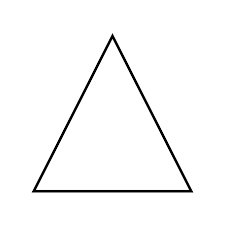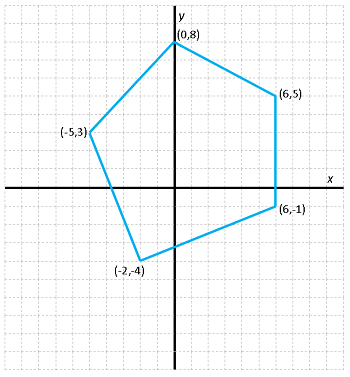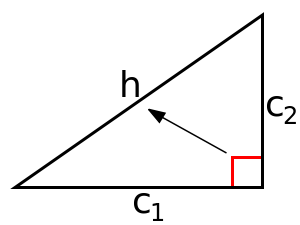# 8th Grade Math Vocabulary Words

Instructor: Artem Cheprasov

Artem has a doctor of veterinary medicine degree.

You're about to go to high school. Oh no, you think, the math classes are going to be really hard, and you don't feel you are totally prepared just yet. In this lesson, you'll learn some of the basic and commonly used vocabulary in your high school math classes, so you can begin your high school career with confidence.

8th grade is a very important time. It's that last year before you enter high school and four years of a more rigorous and challenging curriculum. By the time you get to high school, you want to make sure you know as many important math related words as possible. Why? Look at it this way. if you don't know the words of the English language, you can't read, write, or talk. The same is true for math. If you don't know the words of math, you won't be able to read, write, or speak math. It's kind of hard to do well in school if you aren't prepared.

There are a lot of math words you should know by the end of 8th grade. This lesson can't possibly cover all of them, but you will learn some of the more commonly used math terms.

## Math Words A-M

Let's start with absolute value. This refers to the distance a number is from zero. The absolute value of any number is always positive. The absolute value is written as |x|, where x is a number. For example, the absolute value of 200, |200|, is 200. The absolute value of -200, |-200|, is also 200.

That wasn't too hard and neither is the next term, acute angle. Think of it as being a tiny cute angle. Since it's tiny it has to be less than 90 degrees. Thus, an acute angle is one that is greater than 0 degrees but less than 90 degrees.Angles are sometimes drawn on a coordinate plane. You know, that's the one with the horizontal and vertical axis that intersect at a right angle. In a coordinate plane, an axis is the x or the y line that cross one another at a right angle. In a coordinate plane, each point has a coordinate,a number, denoted as (x,y), that shows the point's position.We can use these coordinates to help rotate figures on a coordinate plane clockwise or counterclockwise. Remember, a clockwise direction refers to the same direction the hands of a clock move while counterclockwise means a movement in the opposite direction.

Shapes don't exist solely on paper, of course. We have three-dimensional shapes, like cubes. But in math, cube refers to more than just a shape. Cube can be taken to mean the third power of some number. For example, if we were to cube 2, the answer would be 8, from 2 x 2 x 2.

You should also know simple terms related to triangles. For example, the hypotenuse is the longest side of a right triangle. The other two sides are called legs. A right triangle is a triangle that has a right angle. In other words, it has a 90-degree angle. The legs are the two sides of a right triangle that actually form this 90-degree angle while the hypotenuse is the side opposite the right angle.To unlock this lesson you must be a Study.com Member.

### Register to view this lesson

Are you a student or a teacher?